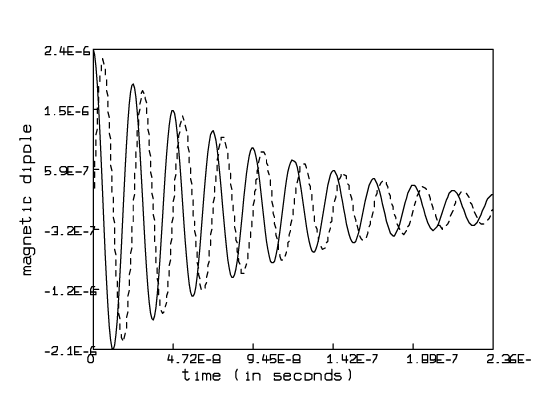## Tuesday, September 20, 2011

### MLAB - Mathematical and statistical modeling

MLAB is an ideal tool for mathematical and statistical exploration, and for solving simulation and modeling problems such as chemical kinetics, pharmacological compartmental models, etc.

MLAB - Mathematical and statistical modeling

Data Analysis and Modeling Services also available.

"Consider the following experiment: a 1 cubic centimeter sample of water at room temperature is placed between the pole faces of a 10,000 Gauss (1 Tesla) electromagnet. As the system reaches equilibrium, a small magnetic dipole of magnitude 3.4E-6 Gauss is induced in the sample parallel to the applied field. "

Free Induction Decay an MLAB ExampleCivilized Software, Inc. 12109 Heritage Park Circle Silver Spring MD 20906 U.S.A.